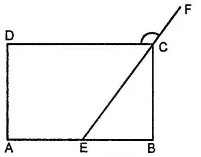Two adjacent angles of a parallelogram have equal measure. Find the measure of each of the angles of the parallelogram.

Asked by Aaryan | 1 year ago |  103

##### Solution :-

Sum of adjacent angles = 180°

∠ A + ∠ B = 180º

2∠ A = 180º (∠ A = ∠ B)

∠ A = 90º

∠ B = ∠ A = 90º

∠ C = ∠ A = 90º (Opposite angles)

∠ D = ∠ B = 90º (Opposite angles)

Thus, each angle of the parallelogram measures 90º.

Answered by Sakshi | 1 year ago

### Related Questions

#### In the given figure, PQRS is a kite. Find the values of x and y.

In the given figure, PQRS is a kite. Find the values of x and y.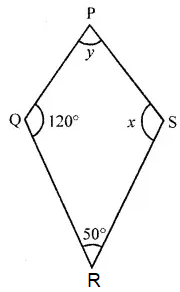#### In the given isosceles trapezium ABCD, ∠C = 102°. Find all the remaining angles of the trapezium.

In the given isosceles trapezium ABCD, ∠C = 102°. Find all the remaining angles of the trapezium.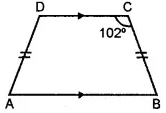#### In the given figure, ABCD is a rhombus and ∠ABD = 50°. Find :

In the given figure, ABCD is a rhombus and ∠ABD = 50°. Find :

(i) ∠CAB

(ii) ∠BCD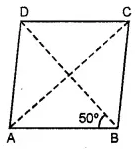#### In the given figure, ABCD is a rectangle and diagonals intersect at O. If ∠AOB = 118°, find

In the given figure, ABCD is a rectangle and diagonals intersect at O. If ∠AOB = 118°, find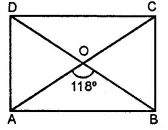(i) ∠ABO

(iii) ∠OCB